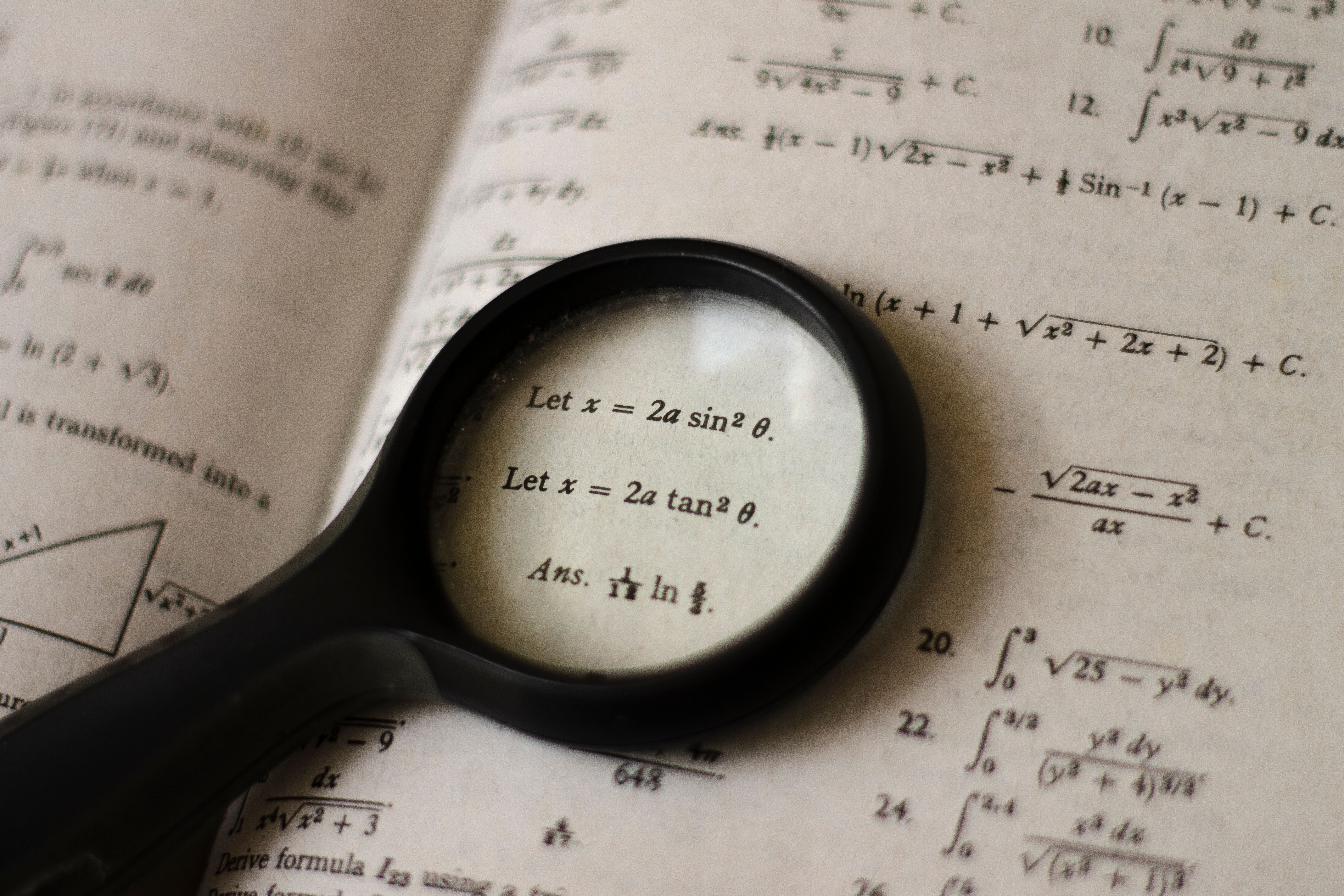# What major is discrete math for?

What <a href="https://www.studentmovementusa.org/is-being-an-econ-major-easy/">Major</a> is Discrete Math For?

# Exploring the Applications of Discrete Math in Computer Science

Majors Applications of Discrete Math in Computer Science
Computer Science Programming languages, software development, cryptography, algorithms
Mathematics Number theory, combinatorics, graph theory, probability theory
Engineering Communication systems, control theory, signal processing

Discrete mathematics is a branch of mathematics that deals with finite, countable sets and the relationships between them. In recent years, it has become increasingly important in many areas of computer science, where it provides a framework for solving complex problems related to computation and data analysis. This article explores the applications of discrete math in computer science and the majors that benefit the most from studying it.

## FAQs About Discrete Math and Its Applications in Computer Science

### What is discrete mathematics?

Discrete mathematics studies mathematical structures that are discrete, meaning that they are finite or countable. This includes topics such as graph theory, combinatorics, set theory, number theory, and probability theory. Unlike continuous mathematics, which deals with continuous, infinite sets, discrete mathematics focuses on solving problems related to computation, algorithms, and data analysis.### What are the applications of discrete math in computer science?

Discrete mathematics has numerous applications in computer science, including:

• Programming languages: Discrete math provides a basis for understanding the syntax and semantics of programming languages. It allows programmers to write efficient, bug-free code that can be easily optimized.
• Software development: Discrete math provides a structured approach to developing software systems that are reliable and can be easily maintained. It helps in the design of databases, networks, and algorithms that underlie software applications.
• Cryptography: Discrete math is used extensively in cryptography to design secure encryption algorithms, digital signatures, and other cryptographic protocols.
• Algorithms: Discrete math provides a framework for designing and analyzing algorithms for solving complex problems. It is used in machine learning, artificial intelligence, and data mining applications to extract patterns and insights from large datasets.### What majors benefit the most from studying discrete math?

Several majors can benefit from studying discrete math, including:

• Computer Science: Discrete math is an essential part of computer science curricula, especially for majors that focus on software development, algorithms, and data analysis. It provides a foundation for many advanced topics in computer science, such as artificial intelligence, computer graphics, and cryptography.
• Mathematics: Discrete math is a fundamental part of mathematics curricula, and it provides a broad range of applications in number theory, graph theory, combinatorics, and probability theory. It is also useful in several areas of physics and engineering.
• Engineering: Discrete math is used in several areas of engineering, including communication systems, control theory, and signal processing. It provides a framework for designing, analyzing, and optimizing these systems.### What are some online resources for learning discrete math?

There are several online resources available for learning discrete math:

• Coursera: Coursera offers several online courses on discrete math, including an introduction to graph theory and a course on combinatorics.
• Khan Academy: Khan Academy offers free online courses on computer science, including several topics related to discrete math such as algorithms, data structures, and cryptography.
• Math Stack Exchange: Math Stack Exchange is an online community of mathematicians, where you can ask questions and get answers related to discrete math, as well as other areas of mathematics.## Conclusion

Discrete mathematics has become increasingly important in computer science, where it provides a foundation for solving complex problems related to computation, algorithms, and data analysis. It includes various topics such as graph theory, set theory, probability theory, and many more. Computer science, mathematics, and engineering are some of the majors that benefit the most from studying discrete math. If you are interested in learning more about discrete math, several online resources are available for free, including Coursera, Khan Academy, and Math Stack Exchange.